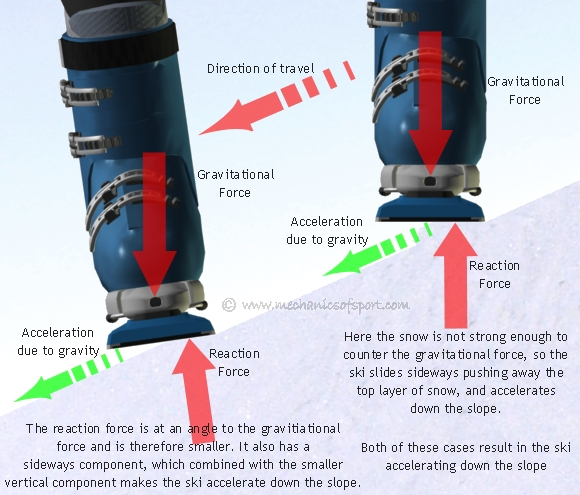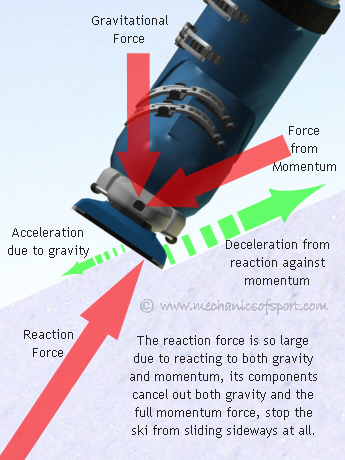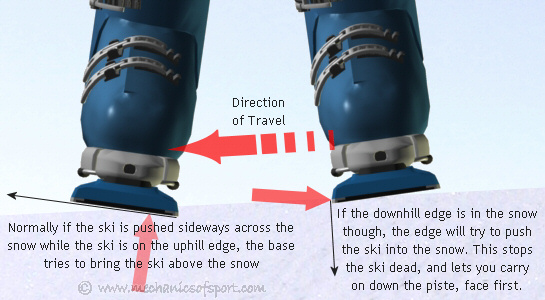SKIING

# Edge Effects

There are 4 different effects an edge can produce as you are skiing, the effects depend on the forces going through the edge, the angle the edge is at, and the snow conditions. Put simply the effects are; make a ski accelerate down a slope, keep a ski at a constant velocity as it travels down a slope, make a ski decelerate as it travels down a slope, and stop a ski so that it doesn't travel any further sideways down a slope. To show how these work to we are going to use the situation where the ski is sideways to a slope, and only consider the sideways forces and movement.

## Making a Ski Accelerate as it Travels Down a Slope

In order for a ski to accelerate sideways as it travels down a slope, the gravitational force must be greater than the vertical component of the reaction force from the snow. There are 2 main ways in which this happens. The ski can be tilted at an angle down the slope, so that the reaction force is not acting in the opposite direction. This means that the vertical component of the reaction force must be smaller than the gravitational force, and that there is a sideways component that will also push the ski down the slope, resulting with the ski accelerating down the slope. The other way is for the ski to be flat to the gravitational force, with the reaction force created acting in the opposite direction to gravity, but with the snow not being strong enough to support the full gravitational force. The snow then gets pushed down the slope by the ski, letting the ski accelerate down the slope.## Making a Ski Slide Down a Slope at a Constant Velocity

For this to happen the reaction force must cancel out the gravitational force and leave no forces left over to cause an acceleration in any direction. Again there are 2 main ways in which this can happen. The first way is very simple, the ski is flat to the gravitational force, with the reaction force is in the opposite direction to gravity, and the snow supporting the ski so that the reaction force is the same magnitude as the gravitational force. This leaves no left over forces and will keep the ski traveling at a constant velocity. The other ways is to have the ski leant up the slope slightly so that the reaction force from the snow has a component that acts sideways up the slope. This component acts as resistance against the skis velocity and makes the skis momentum produce a force. As the reaction force is not vertical the vertical component of the reaction force due to gravity is not strong enough to counteract the gravitational force, but as the ski is opposing momentum as well there is a reaction force due to momentum that needs to be added. To make the ski continue down the slope as a constant speed, the vertical component of the reaction forces due to both gravity and momentum combined cancel out the gravitational force, with the acceleration due to gravity and deceleration due to momentum also canceling each other out.## Making a Ski Decelerate as it Slides Down a Slope

For this to happen the vertical component of the reaction force needs to be greater than the gravitational force, and there to be a sideways force acting up the slope. This will create an acceleration up the slope making the ski decelerate as it travels down the slope. For this to happen the ski is again tilted up the slope making the reaction force oppose both gravity and momentum. This time though the vertical component of the reaction force against momentum is larger than the difference between the gravitational force and the vertical component of the reaction to it. This creates a left over force that acts against momentum, making the ski accelerate up the slope (creates a deceleration) slowing the ski down as it slides.## Making a Ski Stop Dead in the Sideways Direction

In this case, the ski is tilted up the slope again, but this time the reaction force from the snow is so large that both gravity and the full possible force from momentum are both cancelled out completely. This stops all movement in the sideways direction, so the ski does not slide sideways. This is what happens when you either come to a stop, or when you are carving. With carving the ski slides forwards along the edge, having taken all the energy taken from the reaction force and put it into the forwards movement of the ski.Each of these cases can be used in many different ways to give you control, and to be able to ski you need to use all of them. When a ski is on the flat the edges work in the same way, except then gravity can not cause any accelerations down the slope, as there is no slope.

There is one last effect that a ski edge can have, although it still comes into the stopping a ski from moving sideways catergory. It's called catching an edge.

## Catching An Edge

This is a case where resistance can be created in the wrong way. As shown in the diagram below, if the downhill edge touches the snow, it will try to push the ski into the snow, creating a large force against the direction of movement. This stops the ski dead, while your body continues down the slope, as if someone took your legs out (virtually always resulting in a crash). To catch an edge is far more common in snowboarding, but is still possible in skiing. With skis though as you can not move you ankles in ski boots and your knees will not bend sideways, it means that your body has to be quite a long way out of position down the slope, for you to be able to catch an edge. When you do catch an edge though, it is one of the most dangerous parts of skiing, as you will get thrown to the ground harder than in any normal crash.## Digging the Ski into the Snow

There is one more situation that we need to look at, although is being explained last as this is used more with carving, than sliding sideways. This is where the is little or no sideways movement from the ski and the force put through the ski is at a downwards angle to the base. The ski is tilted onto it uphill edge so the reaction force acts at an angle, here though the side of the edge is also pushed into the snow, with another reaction force acting along the side of the edge. The 2 reaction forces together cancel out gravity, so the ski doesn't accelerate, and the sideways forces together stop the ski from sliding. This is used when we want to push the edges into the snow, before trying to put a large force through the base into the snow, or while there is a large force through the base, to keep the ski in the snow. We want to dig the edge into the snow before putting a large force through the base because then the force put into the snow by the base is spread out over a larger area, this puts less pressure into the snow and makes it more likely that the snow will be strong enough to hold the force.In the next section we learn about how to direct the forces we create with the skis, creating more or less resistance, and how these force can be calculated.

On to the Creating Resistance section.